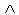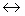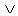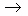Logic
edHelper subscribers - Create a new printable

Number of Keys
 Select the number of different printables: 1 key 2 keys 3 keys 4 keys 5 keys

Number of Pages (for each key)
 Select the number of pages: 1 page 2 pages 3 pages 4 pages

Include an answer key (answer keys will be at the end of the printable)
High School Geometry
High School Geometry
Math

 Name _____________________________Date ___________________
Logic
State the truth value of the following statements.

 1 If p is true and q is false, state the truth for:(~( pq ))(~ p~ q )
 2 If p is true and q is false, state the truth for:(( qp )(~ qp ))(~(~ p~ q ))
 3 If p is false and q is false, state the truth for:( pq )(~(~(~ p~ q )~(~ p~ q )))
 4 If p is false and q is false, state the truth for:(~(~ pq ))(~ p~ q )
 5 If p is false and q is false, state the truth for:( p~ q )([~( pq )])
 6 If p is true and q is false, state the truth for:(( q~ p )~ q )( pq )
 7 If p is true and q is false, state the truth for:(~(~ p~ q ))((~ pq )(~ qp ))
 8 If p is false and q is false, state the truth for:(~ p~ q )(~( pq ))
 9 If p is true and q is true, state the truth for:(~ p~ q )( p~ q )
 10 If p is false and q is false, state the truth for:(~( q~ p )(~ q~ p ))(~(~ pq ))
 11 If p is true and q is false, state the truth for:(~( p(~ q~ p )))(~( p( q~ p )))
 12 If p is true and q is true, state the truth for:( pq )( pq )
 13 If p is true and q is true, state the truth for:( p~ q )( p~ q )
 14 If p is false and q is true, state the truth for:(( pq )(~ qp ))(( qp )q )
 15 If p is false and q is true, state the truth for:(~ pq )(~( qp )(~ pq ))
 16 If p is true and q is true, state the truth for:(( pq )(~ pq ))(~(( p~ q )( q~ p )))

Sample
This is only a sample worksheet.

Logic
edHelper subscribers - Create a new printable

Number of Keys
 Select the number of different printables: 1 key 2 keys 3 keys 4 keys 5 keys

Number of Pages (for each key)
 Select the number of pages: 1 page 2 pages 3 pages 4 pages